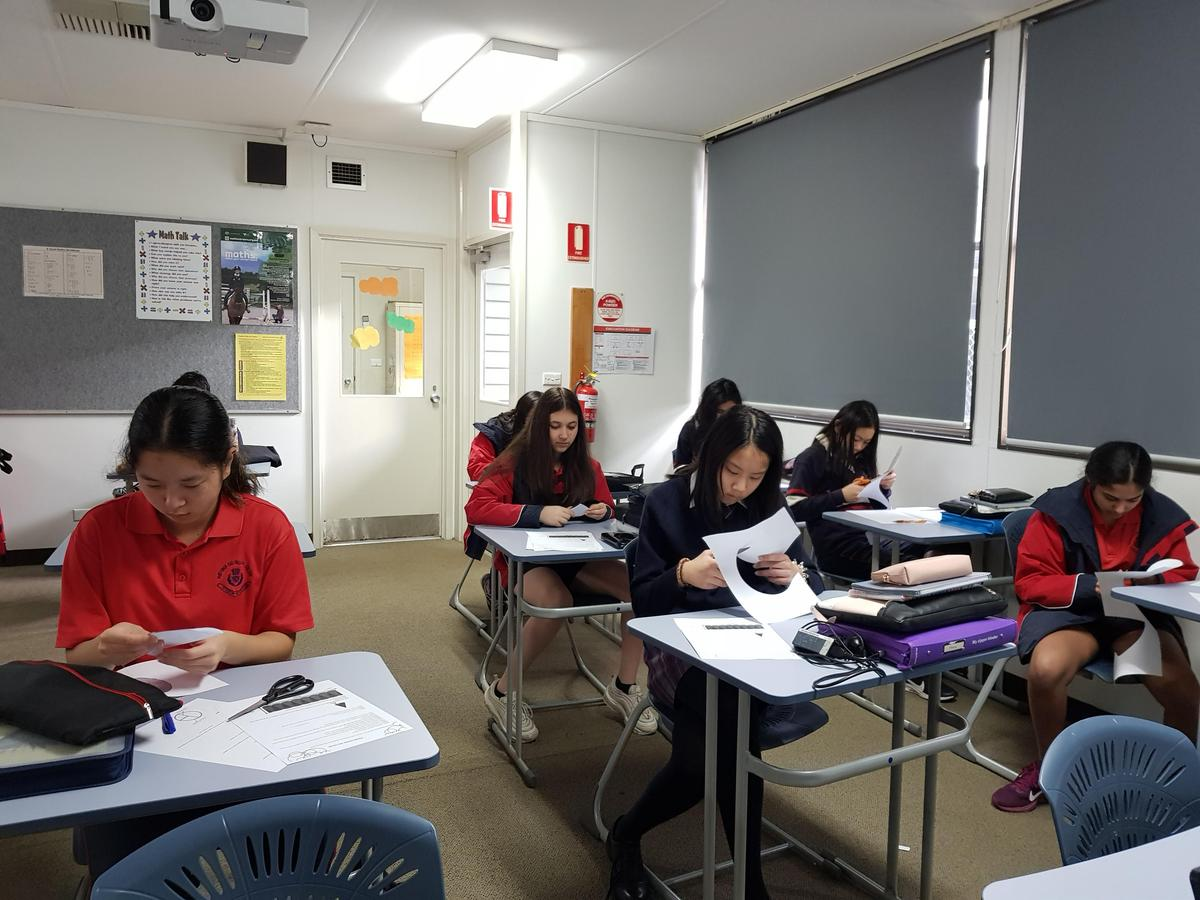# MATHS

## YEAR 8 ELMS MATHS ACTIVITIES

During the last week of term, the Year 8 ELMS students extended our knowledge of measurement, especially the topic of area. The activities we completed enhanced our numerical skills and let us comprehend measurement in a creative yet mathematical way. The first task included the creation of a group of renowned birds and pigs from Angry Birds, which was amusing. The second activity consisted of discovering the relation of circles and rectangles, which required us to ‘think inside the circle’. By the conclusion of these lessons, we had enriched our understanding of measurement with smiles on our faces.

On Tuesday 23 and Wednesday 24 July, we tried a new way of learning maths. Instead of just doing the same workbook questions we did realistic problems finding the total surface area of a 3D shape (a bird) by looking at the net and by understanding why you solve the area of a circle the way you do. Overall this experience was a very enjoyable and unique way of doing maths to get away from repetitive textbook questions.

Ben Green

THINK INSIDE THE CIRCLE

What we did:

After obtaining the worksheets from our teacher, we cut a circle into sixteen sectors, and then we sliced one of the sixteen pieces in half. After arranging the sectors into a specific position, this formed a “rectangle”!

What we learnt:

We learnt that by dividing a circle into infinite pieces, we can frame a rectangle. Therefore, the base of the rectangle is half of the diameter of the circle, while the height of the rectangle is the radius of the circle. This means that the formula of a circle, πr2, is equal to the formula of a rectangle, B x H.

Evie Gallo, Emily Sun, Treya Venkatesh, Nicole Lam & Vernice Koh

I learnt that if you cut the circle up into even parts you can stick them together into a rectangle and the height of the rectangle will be the same as the radius. I also learnt that the length of the base of the rectangle is half of the circumference. The area of the rectangle is also the same area of the circle (if you cut the circle into enough pieces).

Mischa Murray

ANGRY BIRDS SURFACE AREA

Today we measured the total surface area of paper models of Angry Birds. Since different people use different methods to calculate the total surface area, there were many slightly different answers.

Why are there minimal differences between people's measurements? There are many errors that can occur on the way. Such as parallax errors, which is the small difference between the perception of the measurement which can alter the final product. There is expansion with metal rulers as metal contracts with cold and expands with heat. There is also just common human error, as measuring the cutted net or measuring the un cutted, measuring the outside of the black outline or measuring within the black.

Julian Min, Justin Nguyen & Aaron Baker

In this activity we made various birds from Angry Birds using paper nets. Then we all measured the surface area of each shape. Almost everyone got a different result but they were all relatively close. I learned that there are many errors which can happen including a parallax error or a human error. I think that this activity was fun.

Jesse Sumarna